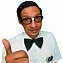@1,1 - cartesian coordinates

Recommended PostsHello,

I was very surprised today. This guy who has been using cad for decades did not know that you can type @ then the coordinates and your new line will reference at the start point of new line and not ucs. Finally, I got him!!! For others, If you did not know that it is something you should check out. I thought that was like universal.

Share on other sitesWhats wrong with line 1,1

Share on other sitesFWIW

See help for Absolute -vs- Relative coordinates.

1,1 or #1,1 is absolute coordinates (use # when DYNamic input is on)

@1,1 is relative coordinates

Share on other sitesI get all these dyn commands confused I think. I put dyn input icon on tray. If I turn it on and draw a line, then a second one off end of first and type 4,4 or #4,4 both go off of origin. If i stick to what i have been doing and type @4,4 it goes 4,4 from endpoint.

Edited by bjenk8100

Share on other sitesUnderstand 4 across 4 up

You might be interested in this could add the 45's say A B C D A4=@4,4

```; draw a pline by direction Up Down left or Right use upper or lowercase
; by Alan H dec 2015
; this version is a metric/decimal version
; version 2 depending on request will be a imperial version

; how to use u123.45 D34.57 R102.6 l53

(defun ah:left ( / pt2)
(setq pt2 (polar pt pi (atof (substr ans 2 (- (strlen ans) 1)))))
(setq pt pt2)
(command pt2)
)

(defun ah:up ( / pt2)
(setq pt2 (polar pt (/ pi 2.0) (atof (substr ans 2 (- (strlen ans) 1)))))
(setq pt pt2)
(command pt2)
)

(defun ah:right ( / pt2)
(setq pt2 (polar pt 0.0 (atof (substr ans 2 (- (strlen ans) 1)))))
(setq pt pt2)
(command pt2)
)

(defun ah:down ( / pt2)
(setq pt2 (polar pt (* 1.5 pi) (atof (substr ans 2 (- (strlen ans) 1)))))
(setq pt pt2)
(command pt2)
)

(defun C:ahpliner ( / pt ans)

(command "_pline")
(command (setq pt (getpoint "Pick start point - Enter or C to finish")))
(while (= (getvar "cmdactive") 1 )
(setq ans (getstring "Enter L123 U456 R67 D78"))
(cond ((= ans "")(command ""))
((= "L"(strcase (substr ans 1 1)))(ah:left))
((= "U"(strcase (substr ans 1 1)))(ah:up))
((= "D"(strcase (substr ans 1 1)))(ah:down))
((= "R"(strcase (substr ans 1 1)))(ah:right))
((= "C"(strcase (substr ans 1 1)))(command "close"))
) ;cond
) ; while
)
```

Edited by BIGAL

Share on other sitesno idea. if i had to do that i would work at walmart instead.

Share on other sitesWhichever is the default is displayed on my status bar as part of my MODEMACRO setting.

```(setvar 'MODEMACRO
(strcat
"\$(If,\$(Eq,\$(Getvar,dbmod),0),,* )" 	;The asterisk indicates the drawing has been modified.
;         "\$(getvar,cprofile)" 				;Current Profile
;         ", \$(getvar,wscurrent)"				;Current Workspace
"\$(getvar,textstyle)"
" \$(getvar,textsize) "
"\$(if,\$(and,1,\$(getvar,pickstyle)),<Group on> ,<Group off> )"
"\$(if,\$(and,16384,\$(getvar,osmode)),,\$(if,\$(and,1,\$(getvar,osmode)),E))"
"\$(if,\$(and,16384,\$(getvar,osmode)),,\$(if,\$(and,2,\$(getvar,osmode)),M))"
"\$(if,\$(and,16384,\$(getvar,osmode)),,\$(if,\$(and,4,\$(getvar,osmode)),C))"
"\$(if,\$(and,16384,\$(getvar,osmode)),,\$(if,\$(and,8,\$(getvar,osmode)),Nod))"
"\$(if,\$(and,16384,\$(getvar,osmode)),,\$(if,\$(and,16,\$(getvar,osmode)),Q))"
"\$(if,\$(and,16384,\$(getvar,osmode)),,\$(if,\$(and,32,\$(getvar,osmode)),Int))"
"\$(if,\$(and,16384,\$(getvar,osmode)),,\$(if,\$(and,4096,\$(getvar,osmode)),Ex))"
"\$(if,\$(and,16384,\$(getvar,osmode)),,\$(if,\$(and,64,\$(getvar,osmode)),Ins))"
"\$(if,\$(and,16384,\$(getvar,osmode)),,\$(if,\$(and,128,\$(getvar,osmode)),Per))"
"\$(if,\$(and,16384,\$(getvar,osmode)),,\$(if,\$(and,256,\$(getvar,osmode)),T))"
"\$(if,\$(and,16384,\$(getvar,osmode)),,\$(if,\$(and,512,\$(getvar,osmode)),Nea))"
"\$(if,\$(and,16384,\$(getvar,osmode)),,\$(if,\$(and,2048,\$(getvar,osmode)),A))"
"\$(if,\$(and,16384,\$(getvar,osmode)),,\$(if,\$(and,8192,\$(getvar,osmode)),Par))"
"\$(if, \$(!=, \$(getvar,viewtwist), 0), Viewtwist: \$(angtos, \$(getvar,viewtwist) [, 0, 2]) , )"	;Thanks alanjt
"\$(if, \$(!=, \$(strlen,\$(getvar,refeditname)), 0), Xref = \$(getvar,refeditname))"
"\$(If,\$(Eq,\$(Getvar,PStyleMode),0),STB,CTB )"
"\$(if,\$(>, \$(getvar,dynmode), 0),\$(if,\$(=,\$(getvar,dynpicoords),0),@,#),#)"	;Dynamic coordinate entry.
" \$(getvar, cmdnames)"
)
)
```

With default setting I toggle Dynamic Mode On/Off with the Status Bar button like rkent.

Share on other sitesI can simplify all that with F12.

Share on other sitesI can simplify all that with F12.

That works same as the Status Bar button with the default setting, but my MODEMACRO setting checks DYNPICOORDS setting as setting it to 1 changes Dynamic Mode to Absolute coordinates.

Share on other sites@ always works for me.

Join the conversation

You can post now and register later. If you have an account, sign in now to post with your account.
Note: Your post will require moderator approval before it will be visible.×   Pasted as rich text.   Paste as plain text instead

Only 75 emoji are allowed.